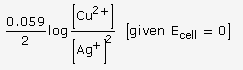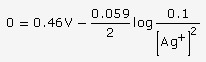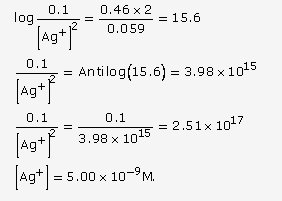# Explain why electrolysis of aqueous solution of NaCl

(a) Explain why electrolysis of aqueous solution of NaCl gives H2 at cathode and Cl2 at anode. Write overall reaction.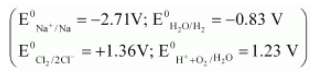(b) Calculate the emf of the cell Zn|Zn2+ (0.1 M) || Cd2+ (0.01 M)|Cd at 298 K,[Given E0Zn2+/Zn = - 0.76 V and E0Cd2+/Cd = - 0.40 V ]

(a) At cathode, the following reduction reactions can take place: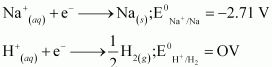A reduction reaction with higher reduction potential is preferred. Therefore, the reaction at the cathode during electrolysis is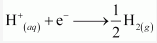This is why electrolysis of aqueous solution of NaCl gives H2 at the cathode.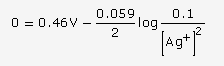At anode, the following oxidation reactions can take place: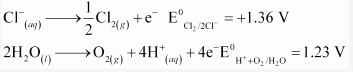At anode, the reaction with lower value of E0 is preferred. But, due to overvoltage, oxidation of chloride ion occurs and chlorine gas is obtained. Hence, the reaction at the anode during electrolysis is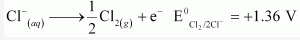This is why electrolysis of aqueous solution of NaCl gives Cl2 at the anode.

The overall cell reaction is given below.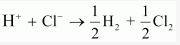(a) Emf of the given cell = Eo cathode - Eoanode
= [- 0.40 - (- 0.76)] V
= [-0.40 + 0.76] V = 0.36 V
Thus, the emf of the given cell is 0.36 V.

or

(a) (i) The alkaline medium prevents the availability of H+ ions. This in turn reduces rate of oxidation of Fe to Fe2+. Thus, the rusting of iron is inhibited.
(ii) Even if the zinc coating is broken in an iron pipe, the remaining zinc layer undergoes oxidation in preference to iron because of its more electropositive nature than iron. Hence iron does not rust.
(b) (i) Since E0Ag+/Ag is greater than EoCu2+/Cu so reduction will occur at silver electrode. The cell representation is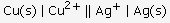(ii) The cell reaction is:
Cu + 2Ag+ (aq)→Clearly, n = 2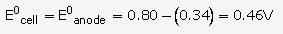At 25oC, Ecell = E0cell -